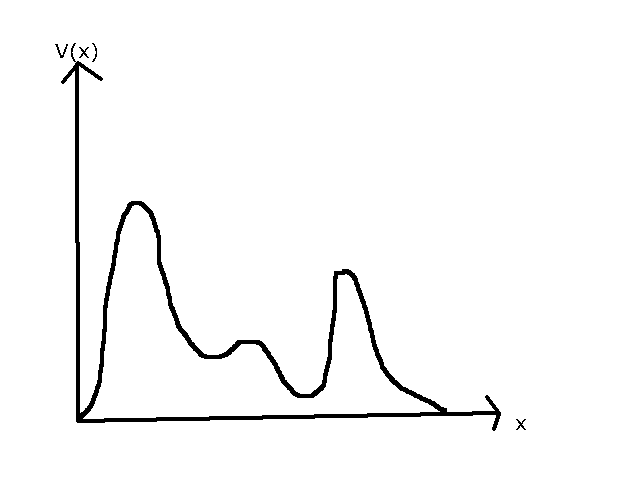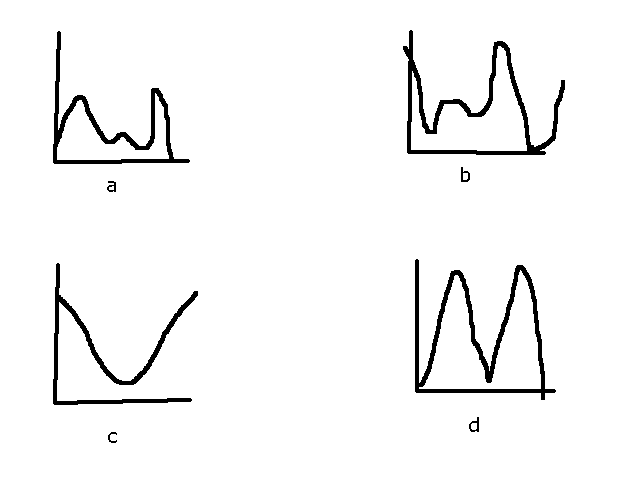# Probability dependence on potential

Homework Statement:
Say, I am given a random looking graph of potential V(x) vs x. I'm not given any information about particle energy. The question then asks me to choose the correct option of ##|\psi (x)|^2##. I feel like the probability density should be higher where potential is lower. But why exactly should that be?
Relevant Equations:
I don't know...
I only know that if E>V, then the frequency would be higher where E-V is higher. But what does that have anything to do with probability?

anuttarasammyak
Gold Member
Shrodinger equation is written as
$$[(\frac{d}{dx})^2+\frac{(E-V)2m}{\hbar^2}]\psi=0$$
Depending on sign of E-V ##\psi## does sinusoidal vibration or exponential dumping.

Yes, I know. I wanted to ask if V is relatively lower at an x, would that imply that the amplitude will be relatively higher?

anuttarasammyak
Gold Member
In order to check your hypothesis why do not you review the cases you well know, e.g. V(x) = kx^2, V(x)=0 for -a<x<a, V otherwise, not only ground state but also excited states ?

For Harmonic oscillator, the ground state is fine. But in the excited states, we can see a piling away from centre.

In the finite square well, if E>V, then the amplitude doesn't change, but frequency decreases in the regions |x|>a.

If E<V, then it shows an exponentially decaying amplitude.

If there's a block of V, then after exponentially decaying, it again becomes simple harmonic with reduced amplitude.

anuttarasammyak
Gold Member
Your consideration seems reasonable to me, though I am not confident in catching your teacher's intention.

kuruman
Homework Helper
Gold Member
Homework Statement:: Say, I am given a random looking graph of potential V(x) vs x. I'm not given any information about particle energy. The question then asks me to choose the correct option of ##|\psi (x)|^2##.
What do you mean by "choose the correct option"? Are you given a certain number of options and are asked to pick one or more? If so, what are they?

The potential is like this:The probability densities are like this:Something like this. c and d are useless. a and b.

a is similar to the potential given. b is the opposite.

kuruman